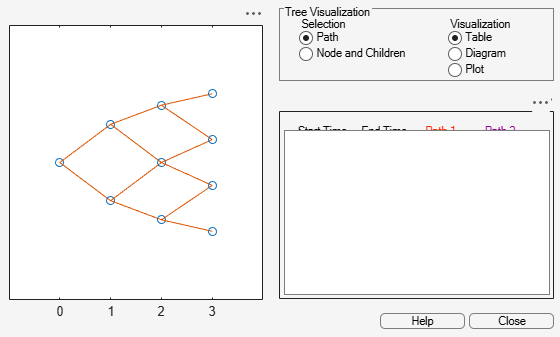# treepath

Entries from node of recombining binomial tree

## Syntax

``Values = treepath(Tree,BranchList)``

## Description

example

````Values = treepath(Tree,BranchList)` extracts entries of a node of a recombining binomial tree. The node path is described by the sequence of branchings taken, starting at the root. The top branch is number one, the second-to-top is two, and so on. Set the branch sequence to zero to obtain the entries at the root node. ```

## Examples

collapse all

Create a `BDT` tree by loading the example file.

```load deriv.mat; FwdRates = treepath(BDTTree.FwdTree, [1 2 1])```
```FwdRates = 4×1 1.1000 1.0979 1.1377 1.1183 ```

This returns the rates at the tree nodes located by taking the up branch, then the down branch, and finally the up branch again.

You can visualize this with the `treeviewer` function.

`treeviewer(BDTTree)`## Input Arguments

collapse all

Recombining binomial tree or trinomial tree specified as a struct that is created using one of the following functions:

Data Types: `struct`

Number of paths (`NUMPATHS`) by path length (`PATHLENGTH`), specified as a matrix containing the sequence of branchings.

Data Types: `double`

## Output Arguments

collapse all

Retrieved entries of a recombining tree, returned a number of values (`NUMVALS`)-by-`NUMPATHS` matrix.

## Version History

Introduced before R2006a